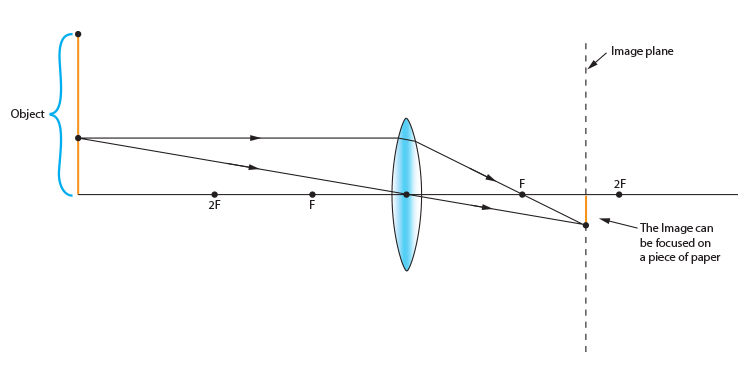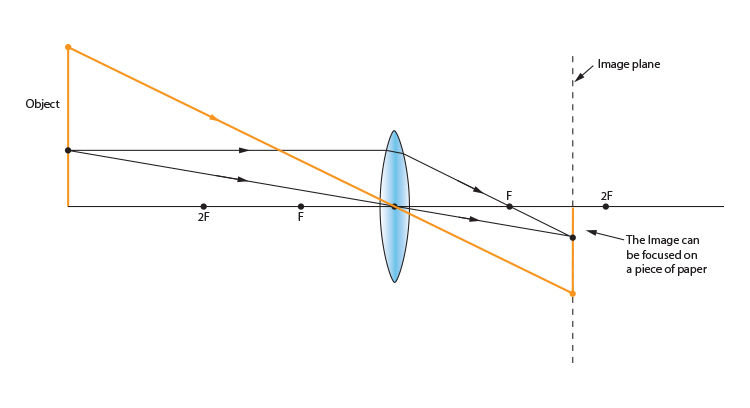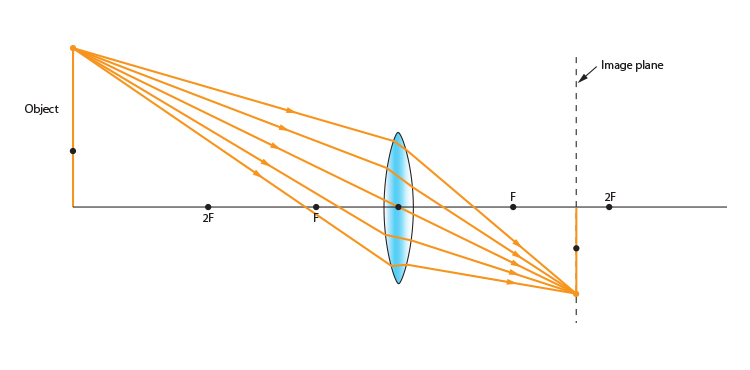# Convex lens and objects larger than the lens

So far, all our objects have been smaller than the convex lens. What happens when the object is larger than the convex lens?

Find out one point on the object that applies to the ray diagram rules and then you have your image plane, as follows:Take two lines from the object. Remember:

1. Pass a parallel line through the principal focal point
2. Pass a ray line through the centre of the convex lens

Now you have the image plane. Draw a line from anywhere on the object through the centre of the convex lens and this will establish the point where it will focus on a piece of paper through the image plane.Now we know where the image top is placed, any other rays can be predicted as follows.